## HELP PLEASE! Write a quadratic function that passes through the point (-1,9), has an axis of symmetry of x=-3 and a minimum value of 7.

Question

HELP PLEASE! Write a quadratic function that passes through the point (-1,9), has an axis of symmetry of x=-3 and a minimum value of 7.

in progress 0
2 months 2021-07-27T17:37:31+00:00 1 Answers 4 views 0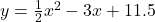Step-by-step explanation:

Vertex Form of a quadratic equation;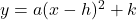Vertex of the parabolas (h, k)

The vertex of the parabola is either the minimum or maximum of the parabola. The axis of symmetry goes through the x-coordinate of the vertex, hence h = -3. The minimum of the parabola is the y-coordinate of the vertex, so k= 7. Now substitute it into the formula;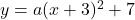Now substitute in the given point; ( -1, 9) and solve for a;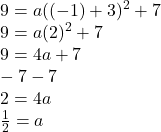Hence the equation in vertex form is;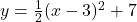In standard form it is;